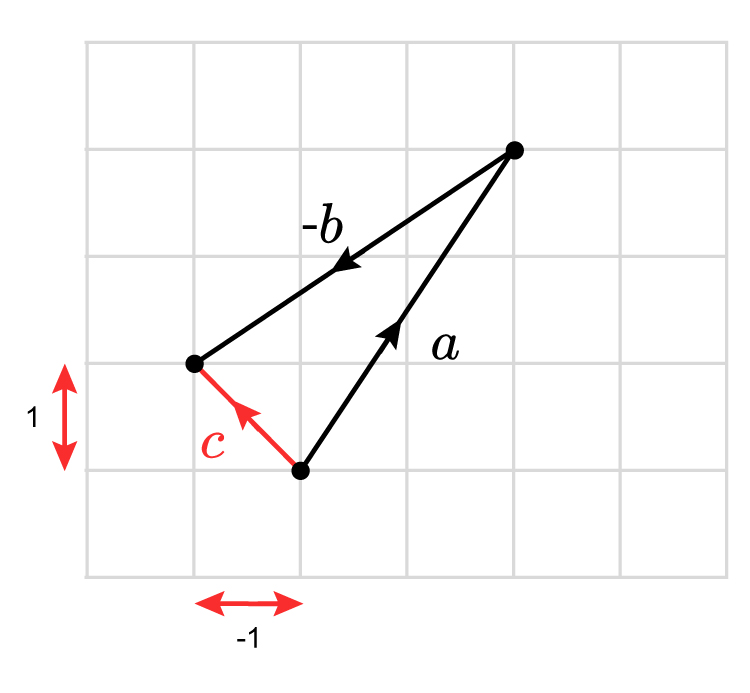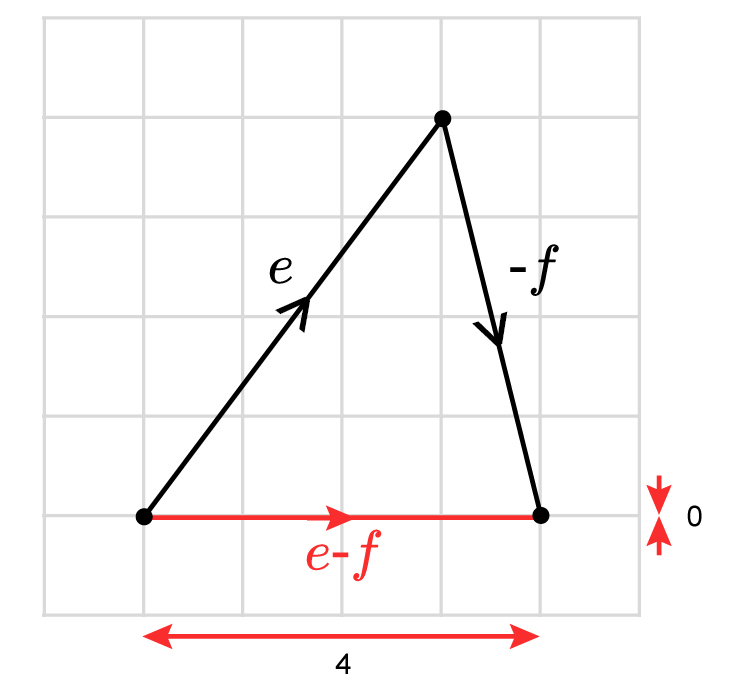# Subtracting column vectors

Subtract just like any normal calculation but keep the top row separate from the bottom row.

Example 1

Take vector a and subtract vector b

vector a=((2),(3))  vector b=((3),(2))

vector a-b=((2-3),(3-2))=((-1),(1))

Shown graphically:vector c=((-1),(1))

Example 2

If vector e=((3),(4)) and vector f=((-1),(4))

What is e-f  ?

((3),(4))-((-1),(4))=((3-(-1)),(4-4))=((4),(0))

Remember two minuses together is a positive.

Shown graphically:e-f=((4),(0))